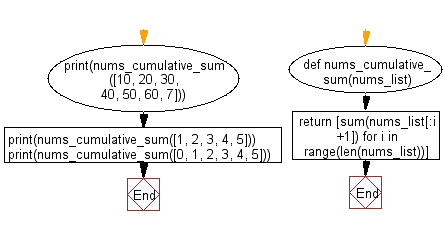﻿ Python: Compute cumulative sum of numbers of a given list - w3resource# Python: Compute cumulative sum of numbers of a given list

## Python Basic - 1: Exercise-92 with Solution

Write a Python program to compute cumulative sum of numbers of a given list.
Note: Cumulative sum = sum of itself + all previous numbers in the said list.

Sample Solution:

Python Code:

``````def nums_cumulative_sum(nums_list):
return [sum(nums_list[:i+1]) for i in range(len(nums_list))]

print(nums_cumulative_sum([10, 20, 30, 40, 50, 60, 7]))
print(nums_cumulative_sum([1, 2, 3, 4, 5]))
print(nums_cumulative_sum([0, 1, 2, 3, 4, 5]))
``````

Sample Output:

```[10, 30, 60, 100, 150, 210, 217]
[1, 3, 6, 10, 15]
[0, 1, 3, 6, 10, 15]
```

Pictorial Presentation:Flowchart:Python Code Editor:

Have another way to solve this solution? Contribute your code (and comments) through Disqus.

What is the difficulty level of this exercise?

Test your Programming skills with w3resource's quiz.

﻿

## Python: Tips of the Day

What is the difference between Python's list methods append and extend?

append: Appends object at the end.

```x = [1, 2, 3]
x.append([4, 5])
print (x)
```

Output:

```[1, 2, 3, [4, 5]]
```

extend: Extends list by appending elements from the iterable.

```x = [1, 2, 3]
x.extend([4, 5])
print (x)
```

Output:

```[1, 2, 3, 4, 5]
```

Ref: https://bit.ly/2AZ6ZFq